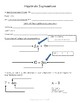# Algebraic Expression Guided NotesSubject
Resource Type
File Type

PDF

(1 MB|35 pages)
Standards
• Product Description
• StandardsNEW

A student friendly guide through Algebraic Expressions! Guided notes include key vocabulary, examples and student practice on the following concepts:

• Parts of the algebraic expression
• Writing algebraic expressions from word phrases
• Writing algebraic expressions from real world situations
• Evaluating algebraic expressions
• Function Tables (Input Output Tables)
• Applying the Distributive Property to write equivalent expressions
• Combining like terms to write equivalent expressions

A variety of types of questions used throughout for student practice: free response, written response, multiple choice, multiple select and error analysis. A final problem solving practice is included combining all concepts.

Identify when two expressions are equivalent (i.e., when the two expressions name the same number regardless of which value is substituted into them). For example, the expressions 𝘺 + 𝘺 + 𝘺 and 3𝘺 are equivalent because they name the same number regardless of which number 𝘺 stands for.
Apply the properties of operations to generate equivalent expressions. For example, apply the distributive property to the expression 3 (2 + 𝘹) to produce the equivalent expression 6 + 3𝘹; apply the distributive property to the expression 24𝘹 + 18𝘺 to produce the equivalent expression 6 (4𝘹 + 3𝘺); apply properties of operations to 𝘺 + 𝘺 + 𝘺 to produce the equivalent expression 3𝘺.
Evaluate expressions at specific values of their variables. Include expressions that arise from formulas used in real-world problems. Perform arithmetic operations, including those involving whole-number exponents, in the conventional order when there are no parentheses to specify a particular order (Order of Operations). For example, use the formulas V = s³ and A = 6 s² to find the volume and surface area of a cube with sides of length s = 1/2.
Identify parts of an expression using mathematical terms (sum, term, product, factor, quotient, coefficient); view one or more parts of an expression as a single entity. For example, describe the expression 2 (8 + 7) as a product of two factors; view (8 + 7) as both a single entity and a sum of two terms.
Write expressions that record operations with numbers and with letters standing for numbers. For example, express the calculation “Subtract 𝘺 from 5” as 5 - 𝘺.
Total Pages
35 pages
Included
Teaching Duration
3 Weeks
Report this Resource to TpT
Reported resources will be reviewed by our team. Report this resource to let us know if this resource violates TpT’s content guidelines.